# Selina Solutions Concise Maths Class 7 Chapter 12: Simple Linear Equations (Including Word Problems) Exercise 12D

Selina Solutions Concise Maths Class 7 Chapter 12 Simple Linear Equations (Including Word Problems) Exercise 12D provides students with a clear idea of solving word problems covered in this chapter. The solutions contain explanations in simple language to make it easy for the students in understanding them. PDFs are available both in exercise and chapter-wise format, based on the requirement of students. Selina Solutions Concise Maths Class 7 Chapter 12 Simple Linear Equations (Including Word Problems) Exercise 12D, free PDF can be downloaded from the links which are provided here.

## Selina Solutions Concise Maths Class 7 Chapter 12: Simple Linear Equations (Including Word Problems) Exercise 12D Download PDF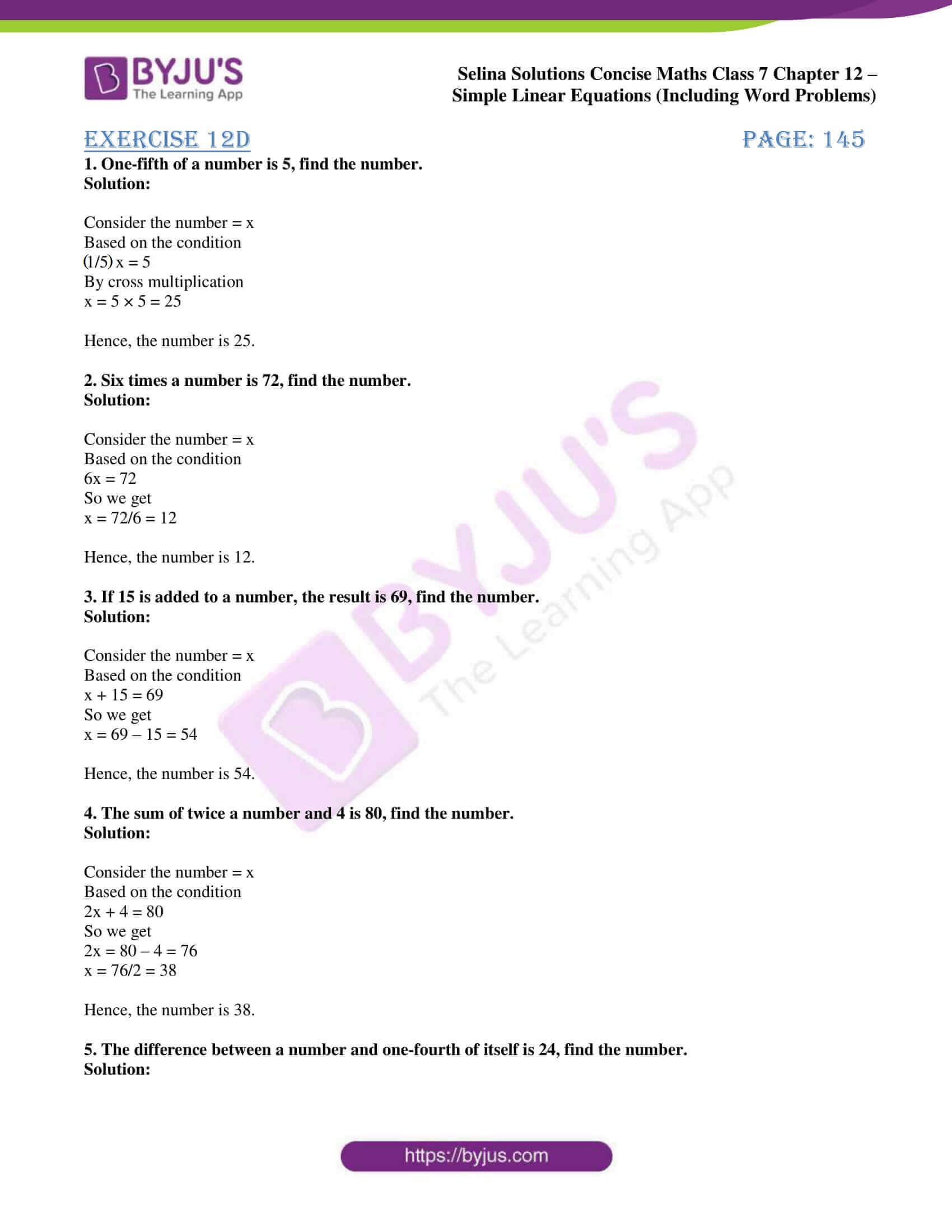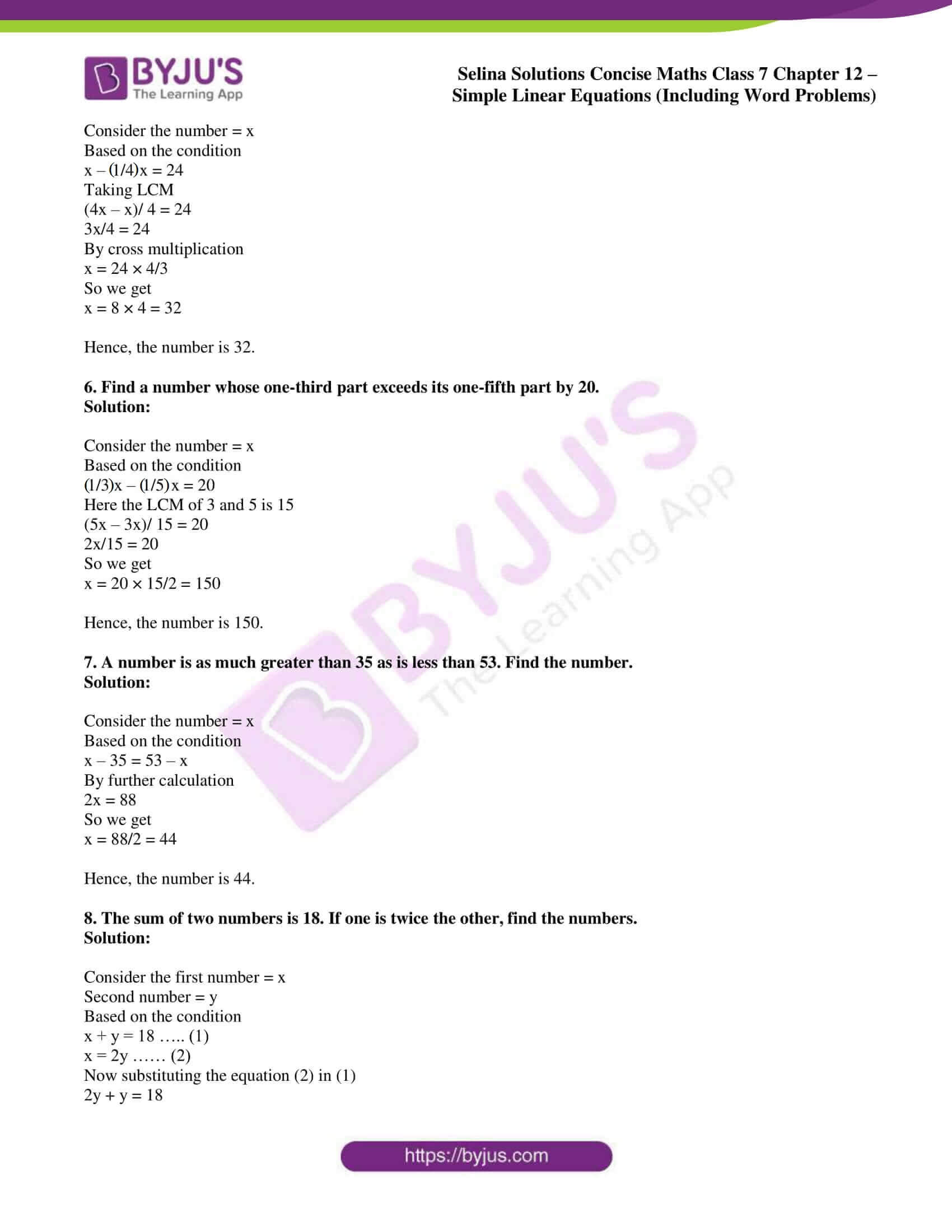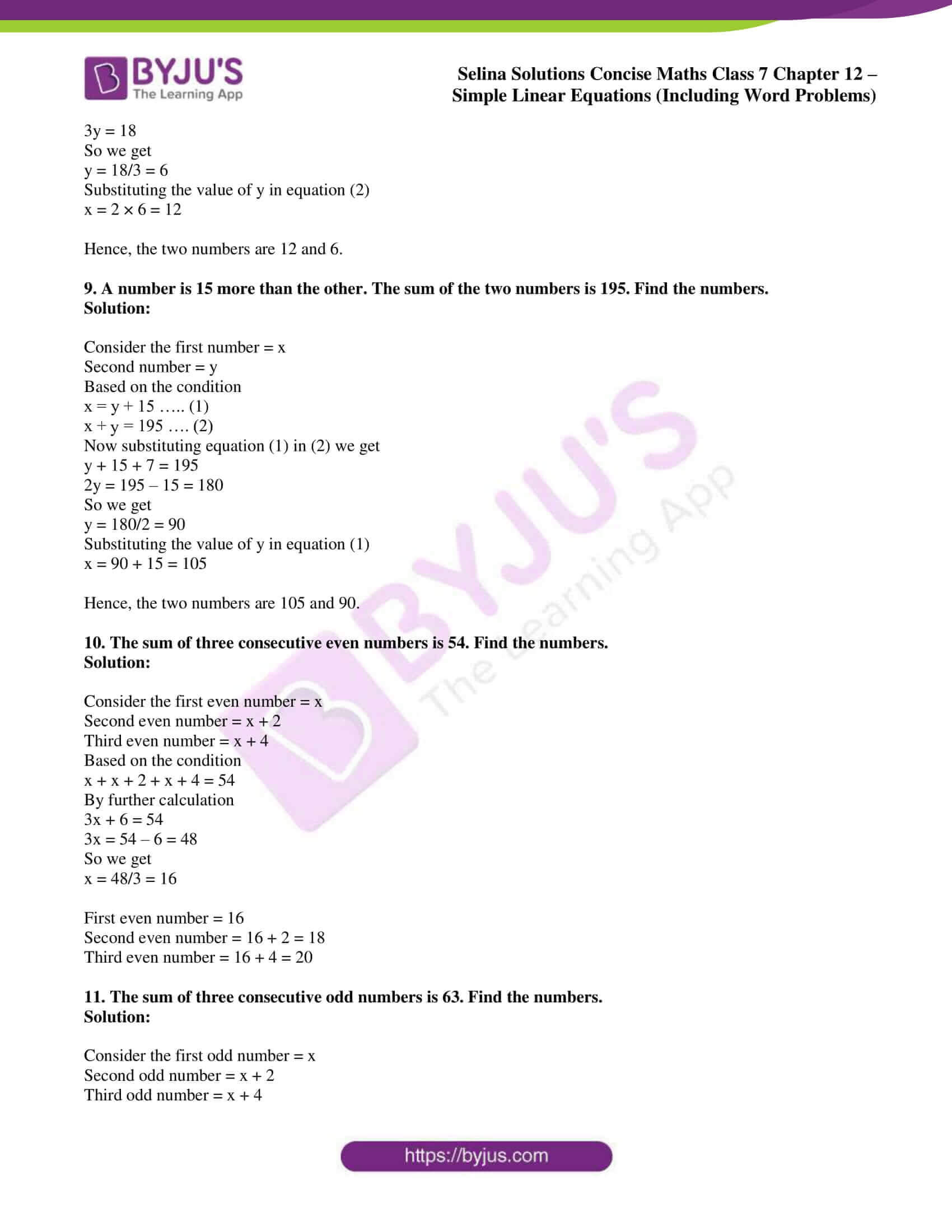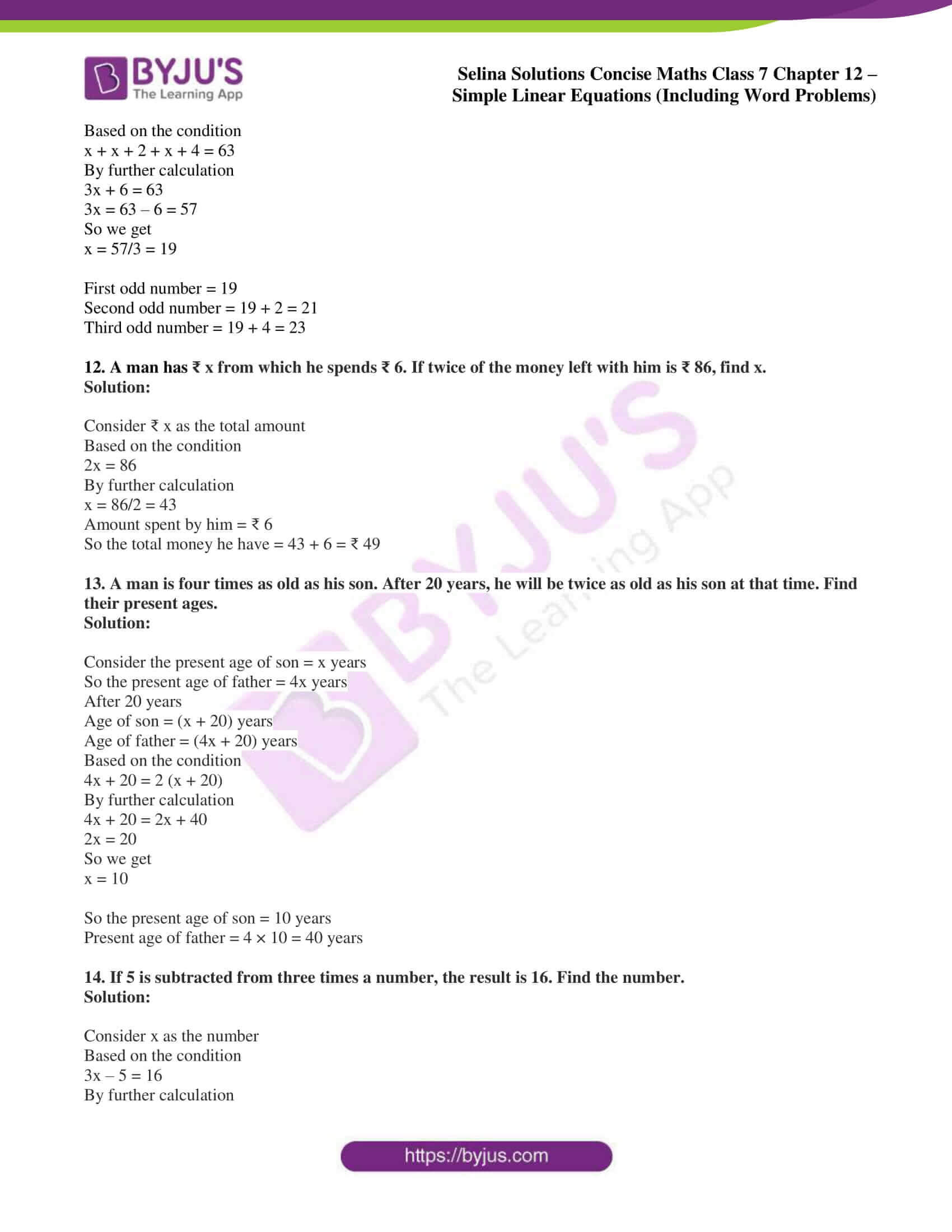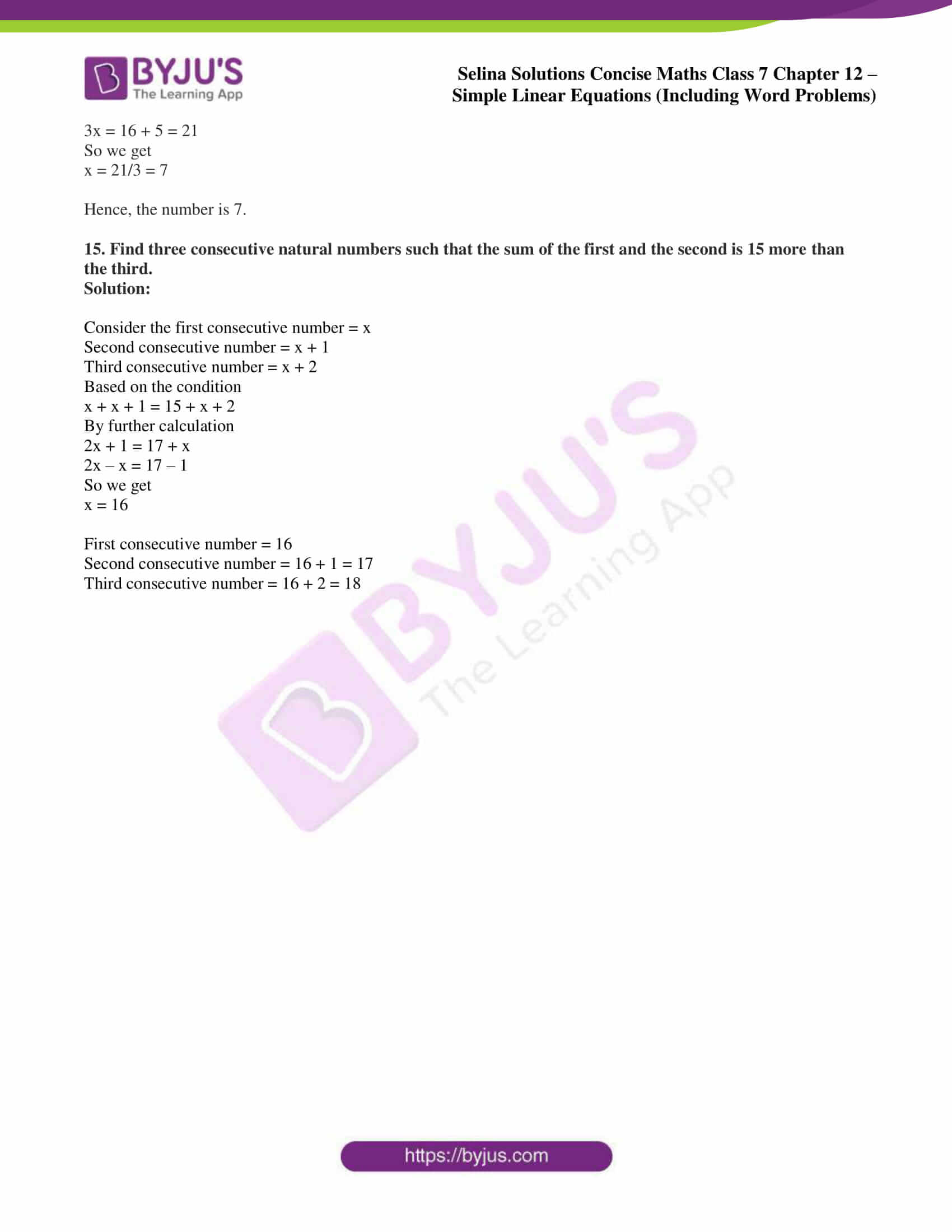### Access other exercises of Selina Solutions Concise Maths Class 7 Chapter 12: Simple Linear Equations (Including Word Problems)

Exercise 12A Solutions

Exercise 12B Solutions

Exercise 12C Solutions

### Access Selina Solutions Concise Maths Class 7 Chapter 12: Simple Linear Equations (Including Word Problems) Exercise 12D

#### Exercise 12D page: 145

1. One-fifth of a number is 5, find the number.

Solution:

Consider the number = x

Based on the condition

(1/5)x = 5

By cross multiplication

x = 5 × 5 = 25

Hence, the number is 25.

2. Six times a number is 72, find the number.

Solution:

Consider the number = x

Based on the condition

6x = 72

So we get

x = 72/6 = 12

Hence, the number is 12.

3. If 15 is added to a number, the result is 69, find the number.

Solution:

Consider the number = x

Based on the condition

x + 15 = 69

So we get

x = 69 – 15 = 54

Hence, the number is 54.

4. The sum of twice a number and 4 is 80, find the number.

Solution:

Consider the number = x

Based on the condition

2x + 4 = 80

So we get

2x = 80 – 4 = 76

x = 76/2 = 38

Hence, the number is 38.

5. The difference between a number and one-fourth of itself is 24, find the number.

Solution:

Consider the number = x

Based on the condition

x – (1/4)x = 24

Taking LCM

(4x – x)/ 4 = 24

3x/4 = 24

By cross multiplication

x = 24 × 4/3

So we get

x = 8 × 4 = 32

Hence, the number is 32.

6. Find a number whose one-third part exceeds its one-fifth part by 20.

Solution:

Consider the number = x

Based on the condition

(1/3)x – (1/5)x = 20

Here the LCM of 3 and 5 is 15

(5x – 3x)/ 15 = 20

2x/15 = 20

So we get

x = 20 × 15/2 = 150

Hence, the number is 150.

7. A number is as much greater than 35 as is less than 53. Find the number.

Solution:

Consider the number = x

Based on the condition

x – 35 = 53 – x

By further calculation

2x = 88

So we get

x = 88/2 = 44

Hence, the number is 44.

8. The sum of two numbers is 18. If one is twice the other, find the numbers.

Solution:

Consider the first number = x

Second number = y

Based on the condition

x + y = 18 ….. (1)

x = 2y …… (2)

Now substituting the equation (2) in (1)

2y + y = 18

3y = 18

So we get

y = 18/3 = 6

Substituting the value of y in equation (2)

x = 2 × 6 = 12

Hence, the two numbers are 12 and 6.

9. A number is 15 more than the other. The sum of the two numbers is 195. Find the numbers.

Solution:

Consider the first number = x

Second number = y

Based on the condition

x = y + 15 ….. (1)

x + y = 195 …. (2)

Now substituting equation (1) in (2) we get

y + 15 + 7 = 195

2y = 195 – 15 = 180

So we get

y = 180/2 = 90

Substituting the value of y in equation (1)

x = 90 + 15 = 105

Hence, the two numbers are 105 and 90.

10. The sum of three consecutive even numbers is 54. Find the numbers.

Solution:

Consider the first even number = x

Second even number = x + 2

Third even number = x + 4

Based on the condition

x + x + 2 + x + 4 = 54

By further calculation

3x + 6 = 54

3x = 54 – 6 = 48

So we get

x = 48/3 = 16

First even number = 16

Second even number = 16 + 2 = 18

Third even number = 16 + 4 = 20

11. The sum of three consecutive odd numbers is 63. Find the numbers.

Solution:

Consider the first odd number = x

Second odd number = x + 2

Third odd number = x + 4

Based on the condition

x + x + 2 + x + 4 = 63

By further calculation

3x + 6 = 63

3x = 63 – 6 = 57

So we get

x = 57/3 = 19

First odd number = 19

Second odd number = 19 + 2 = 21

Third odd number = 19 + 4 = 23

12. A man has ₹ x from which he spends ₹ 6. If twice of the money left with him is ₹ 86, find x.

Solution:

Consider ₹ x as the total amount

Based on the condition

2x = 86

By further calculation

x = 86/2 = 43

Amount spent by him = ₹ 6

So the total money he have = 43 + 6 = ₹ 49

13. A man is four times as old as his son. After 20 years, he will be twice as old as his son at that time. Find their present ages.

Solution:

Consider the present age of son = x years

So the present age of father = 4x years

After 20 years

Age of son = (x + 20) years

Age of father = (4x + 20) years

Based on the condition

4x + 20 = 2 (x + 20)

By further calculation

4x + 20 = 2x + 40

2x = 20

So we get

x = 10

So the present age of son = 10 years

Present age of father = 4 × 10 = 40 years

14. If 5 is subtracted from three times a number, the result is 16. Find the number.

Solution:

Consider x as the number

Based on the condition

3x – 5 = 16

By further calculation

3x = 16 + 5 = 21

So we get

x = 21/3 = 7

Hence, the number is 7.

15. Find three consecutive natural numbers such that the sum of the first and the second is 15 more than the third.

Solution:

Consider the first consecutive number = x

Second consecutive number = x + 1

Third consecutive number = x + 2

Based on the condition

x + x + 1 = 15 + x + 2

By further calculation

2x + 1 = 17 + x

2x – x = 17 – 1

So we get

x = 16

First consecutive number = 16

Second consecutive number = 16 + 1 = 17

Third consecutive number = 16 + 2 = 18